# Overcoming The Nice Guy Syndrome | PUA Archive search results

Home |

## The PUA Archive Search

### Overcoming The Nice Guy Syndrome

```Share or bookmark:<!--
var d=document; if(d.images){ if(!d.Social) d.Social=new Array();
if (a[i].indexOf("#")!=0){ d.Social[j]=new Image; d.Social[j++].src=a[i];}}
}
function schnipp() {
var i,x,a="document.MM_sr;" for(i="0;a&&i<a.length&&(x=a[i])&&x.oSrc;i++)" x.src=x.osrc;
}
function schnupp(n, d) {
var p,i,x; if(!d) d="document;" if((p=n.indexOf("?"))>0&&parent.frames.length) {
d=parent.frames[n.substring(p+1)].document; n=n.substring(0,p);}
if(!(x=d[n])&&d.all) x=d.all[n]; for (i=0;!x&&i<d.forms.length;i++) x=d.forms[i][n];
for(i=0;!x&&d.layers&&i<d.layers.length;i++) x=schnupp(n,d.layers[i].document);
if(!x && d.getelementbyid) x=d.getelementbyid(n); return x;
}
function schnapp() {
var i,j=0,x,a=schnapp.arguments; document.mm_sr=new array; for(i=0;i<(a.length-2);i+=3)
if ((x=schnupp(a[i]))!=null){document.mm_sr[j++]=x; if(!x.osrc) x.osrc=x.src; x.src=a[i+2];}
}
//-->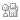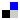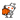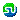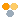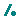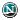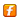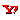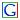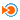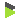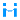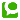Displaying 1 to 1 of 1 matches found, sorted by date.[refine search] [new search]
```

```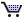Store